Tweeter Mids 8 inch woofer 12 inch woofer 15 inch woofer Built-in Shaker no longer sold

## Beyma

Beyma is a well known producer of speaker in Sevilla, Spain.

## Tweeter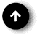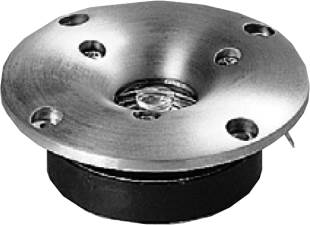T 2030       order no. by-t2030       EUR 59,00excl. VAT: € 49.58 / \$ 56.03
25,4 mm dome tweeter with aluminium dome.
• power handling (continuous/programme) = 15/30 W
• frequency range = 1500-20000 Hz
• resonance frequency fs = 1050 Hz
• impedance R = 8 Ohm
• sound pressure level SPL = 95 dB (2,83V; 1m)
• DC resistance Re = 5 Ohm
• force factor BL = 3 N/A
• total Q factor Qts = 1,122 (Qms=3,957, Qes=1,567)
• voice coil diameter = 25,4 mm
• mounting diameter d = 84,5 mm
• overall diameter d = 102,5 mm
• mounting depth (not countersunk) t = 42 mm
• mass m = 0,7 kg
PDF data sheet

## Midsrecommended cabinet 1:
closed cabinet with 1,3 L volume
from 165/104 Hz (-3dB/-8dB)

recommended cabinet 2:
3,6 L volume bass reflex cabinet
with HP35 reflex tube, 8 cm long.
from 92/71 Hz (-3dB/-8dB).

5 MP 60/N       order no. by-5mp60n       EUR 69,00excl. VAT: € 57.98 / \$ 65.52
12 cm bass-midrange driver with high quality cone.
• power handling (continuous/programme) = 50 W
• frequency range = 50-12000 Hz
• resonance frequency fs = 60 Hz
• impedance R = 8 Ohm
• sound pressure level SPL = 91 dB (2,83V; 1m)
• DC resistance Re = 5,33 Ohm
• voice coil inductance L = 0,4 mH
• effective piston radiating area Sd = 85 cm2
• equivalent volume of compliance Vas = 9,8 l
• total Q factor Qts = 0,29 (Qms=1,604, Qes=0,35)
• voice coil diameter = 26 mm
• maximum peak linear excursion vibration xlin = +/- 4 mm
• mounting diameter d = 120 mm
• overall diameter d = 134 mm
• mounting depth (not countersunk) t = 68 mm
• mass m = 1,2 kg
PDF data sheet5 M 30       order no. by-5m30       EUR 59,00excl. VAT: € 49.58 / \$ 56.03
12 cm midrange driver with paper cone.
• power handling (continuous/programme) = 50/100 W
• frequency range = 60-12000 Hz
• resonance frequency fs = 85 Hz
• impedance R = 8 Ohm
• sound pressure level SPL = 91 dB (2,83V; 1m)
• DC resistance Re = 6,5 Ohm
• force factor BL = 6,4 N/A
• voice coil inductance L = 0,5 mH
• effective piston radiating area Sd = 90 cm2
• effective mechanical mass incl. air load mms = 8 g
• equivalent volume of compliance Vas = 6 l
• total Q factor Qts = 0,61 (Qms=2,72, Qes=0,8)
• voice coil diameter = 25,8 mm
• maximum peak linear excursion vibration xlin = +/- 1 mm
• mounting diameter d = 120 mm
• overall diameter d = 134 mm
• mounting depth (not countersunk) t = 65 mm
• mass m = 1,09 kg
PDF data sheet6 B 30/P       order no. by-6b30p       EUR 57,00excl. VAT: € 47.90 / \$ 54.13
15 cm midrange driver with high quality cone.
• power handling (continuous/programme) = 50 W
• frequency range = 60-10000 Hz
• resonance frequency fs = 55 Hz
• impedance R = 8 Ohm
• sound pressure level SPL = 90 dB (2,83V; 1m)
• DC resistance Re = 5,9 Ohm
• voice coil inductance L = 0,5 mH
• effective piston radiating area Sd = 140 cm2
• equivalent volume of compliance Vas = 18 l
• total Q factor Qts = 0,62 (Qms=3,36, Qes=0,76)
• voice coil diameter = 26 mm
• maximum peak linear excursion vibration xlin = +/- 4 mm
• mounting diameter d = 140 mm
• overall diameter d = 166 mm
• mounting depth (not countersunk) t = 78 mm
• mass m = 1,25 kg
PDF data sheet

8 AG/N       order no. by-8ag-n8       EUR 49,00excl. VAT: € 41.18 / \$ 46.53
19 cm full range driver with paper cone.
• power handling (continuous/programme) = 35 W
• frequency range = 60-18000 Hz
• resonance frequency fs = 101 Hz
• impedance R = 8 Ohm
• sound pressure level SPL = 96 dB (2,83V; 1m)
• DC resistance Re = 7,5 Ohm
• force factor BL = 7,27 N/A
• voice coil inductance L = 0,32 mH
• effective piston radiating area Sd = 227 cm2
• effective mechanical mass incl. air load mms = 14 g
• equivalent volume of compliance Vas = 12,79 l
• total Q factor Qts = 0,98 (Qms=4,45, Qes=1,26)
• voice coil diameter = 35 mm
• mounting diameter d = 180 mm
• overall diameter d = 205 mm
• mounting depth (not countersunk) t = 84 mm
PDF data sheet

## 8 inch wooferopen a bigger photo

recommended cabinet 1:
closed cabinet with 33 L volume
from 55/35 Hz (-3dB/-8dB)

recommended cabinet 2:
134 L volume bass reflex cabinet
with HP100 reflex tube, 14 cm long.
from 26/22 Hz (-3dB/-8dB).

8 WOOFER/P V2       order no. by-8wooferpv2       EUR 89,00excl. VAT: € 74.79 / \$ 84.51
20 cm bass driver with high quality cone.
• power handling (continuous/programme) = 50 W
• frequency range = 30-6500 Hz
• resonance frequency fs = 35 Hz
• impedance R = 8 Ohm
• sound pressure level SPL = 90 dB (2,83V; 1m)
• DC resistance Re = 5,8 Ohm
• voice coil inductance L = 0,9 mH
• effective piston radiating area Sd = 220 cm2
• equivalent volume of compliance Vas = 59 l
• total Q factor Qts = 0,5 (Qms=2,72, Qes=0,62)
• voice coil diameter = 26 mm
• maximum peak linear excursion vibration xlin = +/- 4,5 mm
• mounting diameter d = 182,5 mm
• overall diameter d = 211,5 mm
• mounting depth (not countersunk) t = 76,5 mm
• mass m = 1,3 kg
PDF data sheet

## 12 inch woofer## 15 inch woofer## Built-inHT3100       order no. by-ht3100       EUR 29,00excl. VAT: € 24.37 / \$ 27.54
9 cm coax driver with paper cone.
• power handling (continuous/programme) = 6 W
• frequency range = 100-20000 Hz
• impedance R = 8 Ohm
• sound pressure level SPL = 85 dB (2,83V; 1m)
• mounting diameter d = 91 mm
• overall diameter d = 103,5 mm
• mounting depth (not countersunk) t = 85 mm
• mass m = 0,43 kgIW6       order no. by-iw6       EUR 129,00excl. VAT: € 108.40 / \$ 122.50
cm coax driver with polypropylene cone.
• power handling (continuous/programme) = 50 W
• frequency range = 20-20000 Hz
• impedance R = 8 Ohm
• sound pressure level SPL = 88 dB (2,83V; 1m)
• mounting diameter d = 272x187 mm
• overall diameter d = 308x220 mm
• mounting depth (not countersunk) t = 127 mm
• mass m = 2,6 kg

SE6VT       order no. by-se6vt       EUR 46,00excl. VAT: € 38.66 / \$ 43.68
19 cm coax driver with paper cone.
• power handling (continuous/programme) = 6 W
• frequency range = 60-20000 Hz
• impedance R = 8 Ohm
• sound pressure level SPL = 90 dB (2,83V; 1m)
• mounting diameter d = 181 mm
• overall diameter d = 199 mm
• mounting depth (not countersunk) t = 104 mm
• mass m = 1 kg

## ShakerSK07       order no. by-sk07       EUR 179,00excl. VAT: € 150.42 / \$ 169.97
.
• power handling (continuous/programme) = 50/100 W
• resonance frequency fs = 47 Hz
• impedance R = 16 Ohm
• voice coil diameter = 77 mm
• mass m = 1,5 kg
PDF data sheet

## no longer sold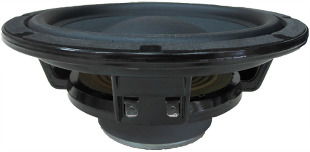open a bigger photo

recommended cabinet 1:
closed cabinet with 54 L volume
from 46/29 Hz (-3dB/-8dB)

recommended cabinet 2:
212 L volume bass reflex cabinet
with HP100 reflex tube, 12 cm long.
from 21/18 Hz (-3dB/-8dB).

8 BR 40/N       order no. by-8br40n       EUR 69,00excl. VAT: € 57.98 / \$ 65.52
20 cm midrange driver with high quality cone.
• power handling (continuous/programme) = 50 W
• frequency range = 35-6000 Hz
• resonance frequency fs = 33,2 Hz
• impedance R = 8 Ohm
• sound pressure level SPL = 90 dB (2,83V; 1m)
• DC resistance Re = 6,5 Ohm
• voice coil inductance L = 0,8 mH
• effective piston radiating area Sd = 220 cm2
• equivalent volume of compliance Vas = 61,35 l
• total Q factor Qts = 0,57 (Qms=2,61, Qes=0,73)
• voice coil diameter = 26 mm
• maximum peak linear excursion vibration xlin = +/- 6 mm
• mounting diameter d = 182,5 mm
• overall diameter d = 211,5 mm
• mounting depth (not countersunk) t = 77,5 mm
• mass m = 1,36 kg
PDF data sheet8 M 60 N       order no. by-8m60n- not available
20 cm midrange driver with paper cone.
• power handling (continuous/programme) = 70/140 W
• frequency range = 120-9000 Hz
• resonance frequency fs = 87 Hz
• impedance R = 8 Ohm
• sound pressure level SPL = 95 dB (2,83V; 1m)
• DC resistance Re = 6,48 Ohm
• force factor BL = 9,1 N/A
• voice coil inductance L = 0,4 mH
• effective piston radiating area Sd = 210 cm2
• effective mechanical mass incl. air load mms = 18 g
• equivalent volume of compliance Vas = 11,3 l
• total Q factor Qts = 0,6 (Qms=2,679, Qes=0,778)
• voice coil diameter = 37,6 mm
• maximum peak linear excursion vibration xlin = +/- 1 mm
• mounting diameter d = 182 mm
• overall diameter d = 214 mm
• mounting depth (not countersunk) t = 86 mm
• mass m = 2,3 kg
PDF data sheetMC 115       order no. by-mc115- not available
44,3 mm mids dome with high quality dome.
• power handling (continuous/programme) = 25 W
• frequency range = 600-12500 Hz
• impedance R = 8 Ohm
• sound pressure level SPL = 91 dB (2,83V; 1m)
• DC resistance Re = 6,13 Ohm
• force factor BL = 7,1 N/A
• total Q factor Qts = 1,127 (Qms=4,894, Qes=1,464)
• voice coil diameter = 44,3 mm
• mounting diameter d = 115 mm
• overall diameter d = 140 mm
• mounting depth (not countersunk) t = 40 mm
• mass m = 1,325 kg
PDF data sheet

CL5T       order no. by-CL5-T- not available
20 cm coax driver with polypropylene cone.
• power handling (continuous/programme) = 30 W
• frequency range = 55-20000 Hz
• impedance R = 8 Ohm
• sound pressure level SPL = 89 dB (2,83V; 1m)
• mounting diameter d = 175 mm
• overall diameter d = 213 mm
• mounting depth (not countersunk) t = 147 mm
• mass m = 1,5 kg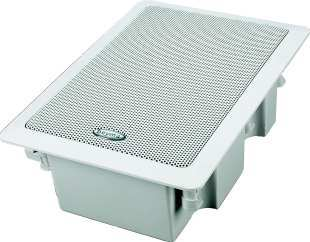IW5       order no. by-iw5- not available
cm coax driver with polypropylene cone.
• power handling (continuous/programme) = 40 W
• frequency range = 20-20000 Hz
• impedance R = 8 Ohm
• sound pressure level SPL = 87 dB (2,83V; 1m)
• mounting diameter d = 264x179 mm
• overall diameter d = 299x214 mm
• mounting depth (not countersunk) t = 115 mm
• mass m = 2 kg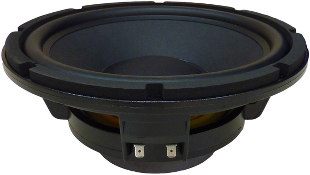open a bigger photo

recommended cabinet 1:
closed cabinet with 21 L volume
from 52/33 Hz (-3dB/-8dB)

recommended cabinet 2:
87 L volume bass reflex cabinet
with HP100 reflex tube, 30 cm long.
from 24/20 Hz (-3dB/-8dB).

10 BR 60 V2       order no. by-10br60v2       EUR 114,00excl. VAT: € 95.80 / \$ 108.25
25 cm bass driver with high quality cone.
• power handling (continuous/programme) = 100/200 W
• frequency range = 30-5000 Hz
• resonance frequency fs = 31 Hz
• impedance R = 8 Ohm
• sound pressure level SPL = 90,6 dB (2,83V; 1m)
• DC resistance Re = 6,5 Ohm
• voice coil inductance L = 1,5 mH
• effective piston radiating area Sd = 380 cm2
• equivalent volume of compliance Vas = 47 l
• total Q factor Qts = 0,47 (Qms=3,29, Qes=0,55)
• voice coil diameter = 52 mm
• maximum peak linear excursion vibration xlin = +/- 6,5 mm
• mounting diameter d = 232 mm
• overall diameter d = 260,5 mm
• mounting depth (not countersunk) t = 111 mm
• mass m = 2,87 kg
PDF data sheetrecommended cabinet 1:
closed cabinet with 20 L volume
from 77/49 Hz (-3dB/-8dB)

recommended cabinet 2:
82 L volume bass reflex cabinet
with HP100 reflex tube, 11 cm long.
from 36/30 Hz (-3dB/-8dB).

10 BR 60       order no. not-available- not available
26 cm midrange driver with high quality cone.
• power handling (continuous/programme) = 100 W
• frequency range = 30-5000 Hz
• resonance frequency fs = 45 Hz
• impedance R = 8 Ohm
• sound pressure level SPL = 91,5 dB (2,83V; 1m)
• DC resistance Re = 5,6 Ohm
• voice coil inductance L = 1,1 mH
• effective piston radiating area Sd = 380 cm2
• equivalent volume of compliance Vas = 47 l
• total Q factor Qts = 0,47 (Qms=1,9, Qes=0,62)
• voice coil diameter = 52 mm
• maximum peak linear excursion vibration xlin = +/- 6 mm
• mounting diameter d = 240 mm
• overall diameter d = 270 mm
• mounting depth (not countersunk) t = 111 mm
PDF data sheet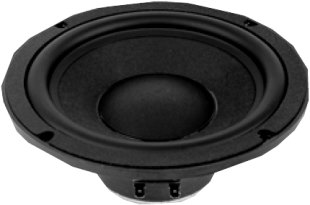recommended cabinet 1:
closed cabinet with 37 L volume
from 52/33 Hz (-3dB/-8dB)

recommended cabinet 2:
152 L volume bass reflex cabinet
with HP100 reflex tube, 14 cm long.
from 24/20 Hz (-3dB/-8dB).

8 BR 40       order no. not-available- not available
20 cm midrange driver with high quality cone.
• power handling (continuous/programme) = 50 W
• frequency range = 35-6000 Hz
• resonance frequency fs = 31 Hz
• impedance R = 8 Ohm
• sound pressure level SPL = 90 dB (2,83V; 1m)
• DC resistance Re = 6,3 Ohm
• voice coil inductance L = 0,7 mH
• effective piston radiating area Sd = 220 cm2
• equivalent volume of compliance Vas = 82 l
• total Q factor Qts = 0,48 (Qms=2,29, Qes=0,6)
• voice coil diameter = 26 mm
• maximum peak linear excursion vibration xlin = +/- 6 mm
• mounting diameter d = 182 mm
• overall diameter d = 216 mm
• mounting depth (not countersunk) t = 83 mm
PDF data sheet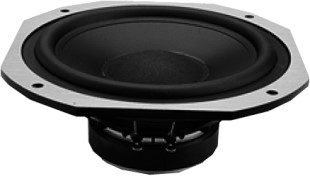recommended cabinet 1:
closed cabinet with 19 L volume
from 72/45 Hz (-3dB/-8dB)

recommended cabinet 2:
71 L volume bass reflex cabinet
with HP100 reflex tube, 17 cm long.
from 36/29 Hz (-3dB/-8dB).

8 WOOFER/P       order no. not-available- not available
20 cm bass driver with paper cone.
• power handling (continuous/programme) = 50 W
• frequency range = 30-3500 Hz
• resonance frequency fs = 35 Hz
• impedance R = 8 Ohm
• sound pressure level SPL = 92 dB (2,83V; 1m)
• DC resistance Re = 5,37 Ohm
• voice coil inductance L = 0,6 mH
• effective piston radiating area Sd = 220 cm2
• equivalent volume of compliance Vas = 75 l
• total Q factor Qts = 0,39 (Qms=2,33, Qes=0,47)
• voice coil diameter = 26 mm
• maximum peak linear excursion vibration xlin = +/- 4,5 mm
• mounting diameter d = 182 mm
• overall diameter d = 214 mm
• mounting depth (not countersunk) t = 86 mm
PDF data sheet

8 BX Koaxial       order no. not-available- not available
20 cm coax driver with polypropylene cone.
• power handling (continuous/programme) = 100/200 W
• frequency range = 25-20000 Hz
• resonance frequency fs = 52 Hz
• impedance R = 8 Ohm
• sound pressure level SPL = 92 dB (2,83V; 1m)
• DC resistance Re = 5,5 Ohm
• force factor BL = 7,3 N/A
• voice coil inductance L = 0,2 mH
• effective piston radiating area Sd = 220 cm2
• effective mechanical mass incl. air load mms = 23 g
• equivalent volume of compliance Vas = 23 l
• total Q factor Qts = 0,68 (Qms=6,1, Qes=0,77)
• voice coil diameter = 38,5 mm
• maximum peak linear excursion vibration xlin = +/- 5 mm
• mounting diameter d = 182 mm
• overall diameter d = 216 mm
• mounting depth (not countersunk) t = 120 mm
• mass m = 2,9 kg
PDF data sheet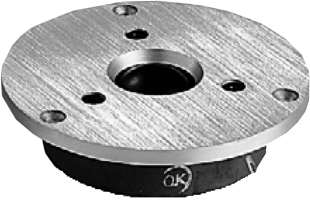T 2010       order no. not-available- not available
25,8 mm dome tweeter with high quality dome.
• power handling (continuous/programme) = 12/24 W
• frequency range = 1500-20000 Hz
• impedance R = 8 Ohm
• sound pressure level SPL = 92 dB (2,83V; 1m)
• DC resistance Re = 6 Ohm
• force factor BL = 3,2 N/A
• total Q factor Qts = 1,638 (Qms=5,185, Qes=2,394)
• voice coil diameter = 25,8 mm
• mounting diameter d = 84,5 mm
• overall diameter d = 100 mm
• mounting depth (not countersunk) t = 29 mm
• mass m = 0,6 kg
PDF data sheet

CL6T       order no. not-available- not available
22 cm coax driver with polypropylene cone.
• power handling (continuous/programme) = 45 W
• frequency range = 45-20000 Hz
• impedance R = 8 Ohm
• sound pressure level SPL = 89 dB (2,83V; 1m)
• mounting diameter d = 205 mm
• overall diameter d = 239 mm
• mounting depth (not countersunk) t = 172 mm
• mass m = 2 kg
PDF data sheet

CL8T       order no. not-available- not available
26 cm coax driver with polypropylene cone.
• power handling (continuous/programme) = 55 W
• frequency range = 40-20000 Hz
• impedance R = 8 Ohm
• sound pressure level SPL = 91 dB (2,83V; 1m)
• mounting diameter d = 245 mm
• overall diameter d = 280 mm
• mounting depth (not countersunk) t = 210 mm
• mass m = 2,8 kg
PDF data sheetrecommended cabinet 1:
closed cabinet with 32 L volume
from 78/49 Hz (-3dB/-8dB)

recommended cabinet 2:
112 L volume bass reflex cabinet
with 2x HP100 reflex tube, 15 cm long.
from 40/32 Hz (-3dB/-8dB).

12 BR 70       order no. by-12br70       EUR 119,00excl. VAT: € 100.00 / \$ 113.00
30 cm bass driver with high quality cone.
• power handling (continuous/programme) = 125 W
• frequency range = 25-2500 Hz
• resonance frequency fs = 35 Hz
• impedance R = 8 Ohm
• sound pressure level SPL = 94,5 dB (2,83V; 1m)
• DC resistance Re = 5,6 Ohm
• voice coil inductance L = 1 mH
• effective piston radiating area Sd = 540 cm2
• equivalent volume of compliance Vas = 154 l
• total Q factor Qts = 0,36 (Qms=1,85, Qes=0,44)
• voice coil diameter = 52 mm
• maximum peak linear excursion vibration xlin = +/- 6 mm
• mounting diameter d = 286 mm
• overall diameter d = 320 mm
• mounting depth (not countersunk) t = 125 mm
PDF data sheetrecommended cabinet 1:
closed cabinet with 21 L volume
from 77/49 Hz (-3dB/-8dB)

recommended cabinet 2:
62 L volume bass reflex cabinet
with 2x HP100 reflex tube, 36 cm long.
from 43/33 Hz (-3dB/-8dB).

12 B 100/R       order no. by-12b100r- not available
30 cm bass driver with high quality cone.
• power handling (continuous/programme) = 150 W
• frequency range = 25-2500 Hz
• resonance frequency fs = 29 Hz
• impedance R = 8 Ohm
• sound pressure level SPL = 93,5 dB (2,83V; 1m)
• DC resistance Re = 6,6 Ohm
• voice coil inductance L = 1,3 mH
• effective piston radiating area Sd = 530 cm2
• equivalent volume of compliance Vas = 154 l
• total Q factor Qts = 0,3 (Qms=6,49, Qes=0,31)
• voice coil diameter = 100 mm
• maximum peak linear excursion vibration xlin = +/- 3 mm
• mounting diameter d = 286 mm
• overall diameter d = 320 mm
• mounting depth (not countersunk) t = 125 mm
PDF data sheetopen a bigger photo

recommended cabinet 1:
closed cabinet with 23 L volume
from 95/60 Hz (-3dB/-8dB)

recommended cabinet 2:
56 L volume bass reflex cabinet
with 2x HP100 reflex tube, 24 cm long.
from 57/43 Hz (-3dB/-8dB).

15 B 100R/GH       order no. by-15b100rgh       EUR 349,00excl. VAT: € 293.28 / \$ 331.40
37 cm bass driver with high quality cone.
• power handling (continuous/programme) = 400/800 W
• frequency range = 40-4500 Hz
• resonance frequency fs = 30 Hz
• impedance R = 8 Ohm
• sound pressure level SPL = 98 dB (2,83V; 1m)
• DC resistance Re = 5,4 Ohm
• force factor BL = 21,8 N/A
• voice coil inductance L = 1,6 mH
• effective piston radiating area Sd = 880 cm2
• effective mechanical mass incl. air load mms = 119 g
• equivalent volume of compliance Vas = 249 l
• total Q factor Qts = 0,25 (Qms=10,894, Qes=0,258)
• voice coil diameter = 100 mm
• maximum peak linear excursion vibration xlin = +/- 6 mm
• mounting diameter d = 355 mm
• overall diameter d = 388 mm
• mounting depth (not countersunk) t = 145 mm
PDF data sheet

 Tweeter Mids 8 inch woofer 12 inch woofer 15 inch woofer Built-in Shaker no longer sold
The cabinet volume calculations are executed considering an external resistance of 0.3 Ohm. We are using, if no other data is known or available, the information supplied by the manufacturer.

Please note: the frequency response doesn't say much about the cut-off frequency inside the cabinet! Therefore, we mostly give you 2 examples with dimensioning considerations and an indication, how low the loudspeaker will reproduce.

Home